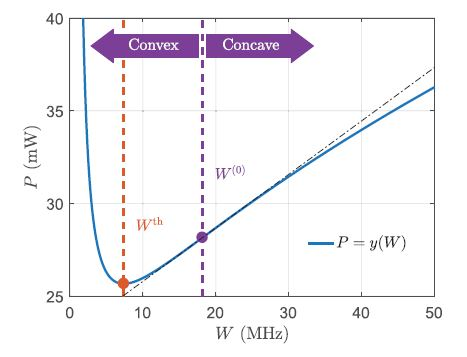# Help! How to rebuild my model with DCP rules

Hello, I am trying to solve this convex proble, I am stuck in the object function. How to rewrite it to the form which cvx accepts?

variables are Wu and Wd, other parameters are known, function y(W) is convex in a part of region, which is proved in the paper. In this problem, W is constrained within the convex region.

When I write term "wdexp[]", there is an error saying Cannot perform the operation: {real affine} . {convex}, wd is affine, exp[] is convex. How can I solve this?
I try to use exp(log(wd))*exp[], it becomes exp(log(wd)+[]), there is still an error saying can’t perform the operation concave+convex. exp(log(wd)) is concave.

Thank you Mark! Anyway I know I might need to seek other methods.But I still want to check with you Mark, this figure is a demonstration of y(W), it is convex in (0,W0), the constraints of W is W belongs to (0, Wth), in this case, it is not possible to solve using cvx, correct? What about transform it into GP form? or yalmip?